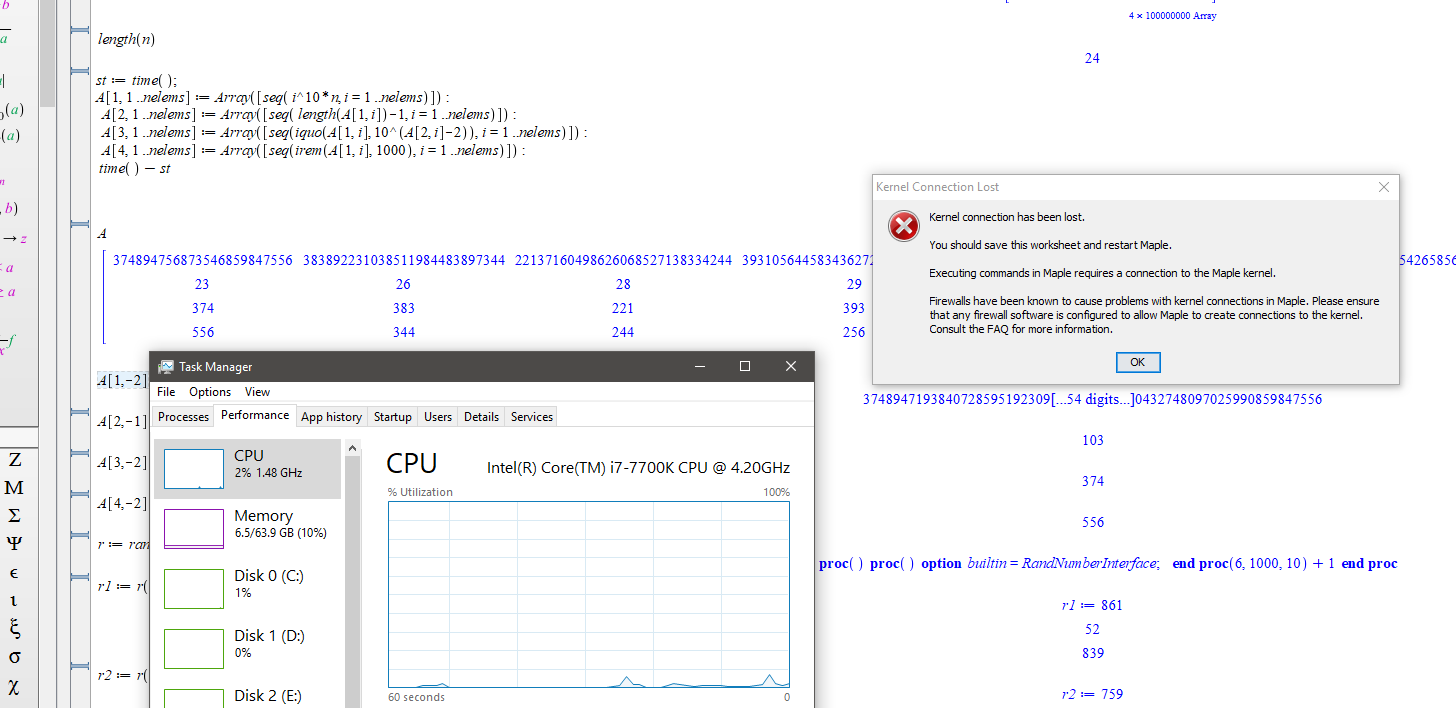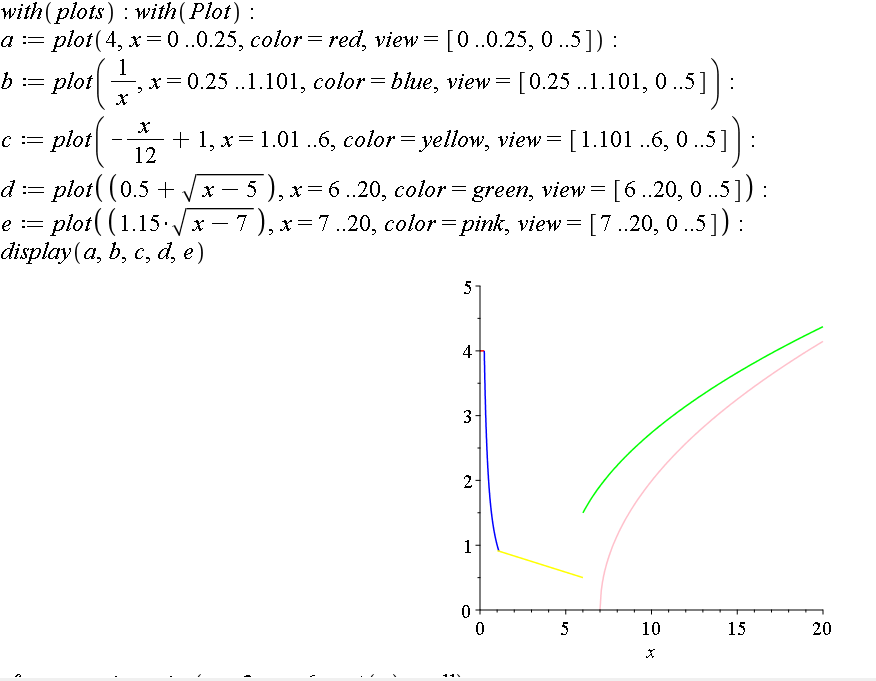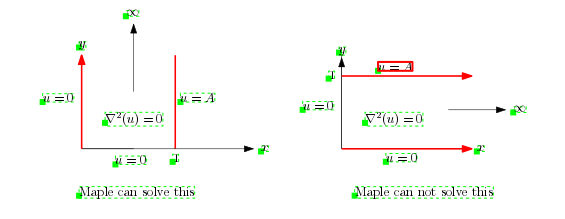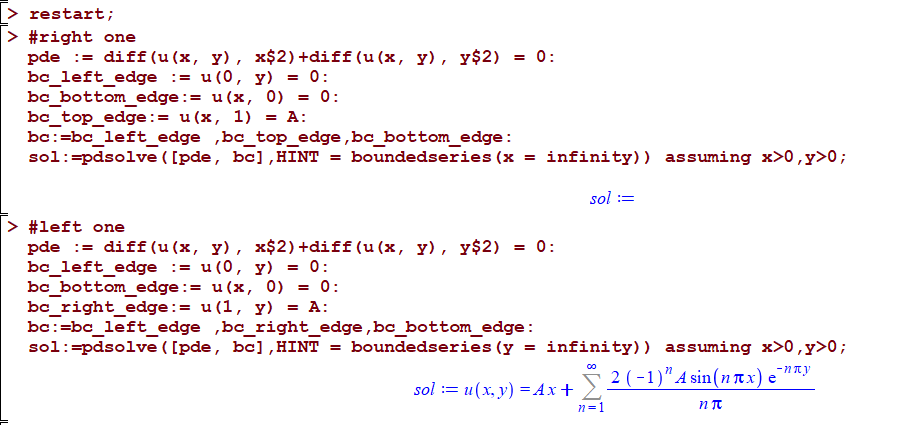## Odditity in Maple 2019...

I have noticed a few times now with Maple 2019. It looses kernel connection when it is sitting there idly. This time I observed it. Had saved a document after an intensive calculation. The memory used was about 30Gig. shortly after saving the cpu fan was running hard. I checked task manager and cpu was cycling to 100%, it was mserever. Then the memory usage droped to about 6gig and message as shown. During this time Maple screen down in the LH corner displayed "Ready", so it didn't think it was doing anything.## Can seq or Seq be applied to fill our a 2 d Array?...

My code below works but I an looking to speed it up to apply to large arrays. I have large integers and I want to store the exponent and first and last few digits.

```nelems := 10;  ~ takes about 50mins when n=100,000,000
n := 374894756873546859847556;
op(n);
A := Array(1 .. 4, 1 .. nelems);
length(n);
st := time();
for i to nelems do
A[1, i] := i^10*n;
A[2, i] := length(A[1, i]) - 1;
b := convert(A[1, i], string);
A[3, i] := parse(b[1 .. 3]);
A[4, i] := parse(b[-3 .. -1]);
end do;
time() - st;
A;
A[1, -2];
A[2, -2];
A[3, -2];
A[4, -2];
```

## simplify equation ...

Hello,

How I can take variation from left-hand side of  5, and reach to right-hand side of  5. After by using integral by part obtained  7?

Thank you## Multiple plots in maple...

Hello,

I´a currently making a school assigment, but i can´t get maple plots to combine the graphs i plot, when i plot multiple plots. (see picture) (the code is in the bottom of the question)as you can see the green and yellow grafh won´t combine. I have tried google but couldn´t find any anserws that worked.
Hope someone can help me!
Thanks!

with(plots); with(Plot);
a := plot(4, x = 0 .. .25, color = red, view = [0 .. .25, 0 .. 5]);
b := plot(1/x, x = .25 .. 1.101, color = blue, view = [.25 .. 1.101, 0 .. 5]);
c := plot(-(1/12)*x+1, x = 1.01 .. 6, color = yellow, view = [1.101 .. 6, 0 .. 5]);
d := plot(.5+sqrt(x-5), x = 6 .. 20, color = green, view = [6 .. 20, 0 .. 5]);
e := plot(1.15*sqrt(x-7), x = 7 .. 20, color = pink, view = [7 .. 20, 0 .. 5]);
display(a, b, c, d, e);

## pdsolve and laplace PDE on semi-infinite domain...

Hello Maple experts;

I am not able to understand why Maple 2019 can solve Laplace PDE in 2D Catersian on semi-infinite domain, when the infinity is along the Y direction, but not along the X direction, since the solution method is exactly the same.Here is the code

```restart;

#right one, Maple can not solve
pde := diff(u(x, y), x\$2)+diff(u(x, y), y\$2) = 0:
bc_left_edge := u(0, y) = 0:
bc_bottom_edge:= u(x, 0) = 0:
bc_top_edge:= u(x, 1) = A:
bc:=bc_left_edge ,bc_top_edge,bc_bottom_edge:
sol:=pdsolve([pde, bc],HINT = boundedseries(x = infinity)) assuming x>0,y>0;

#left one, Maple can solve
pde := diff(u(x, y), x\$2)+diff(u(x, y), y\$2) = 0:
bc_left_edge := u(0, y) = 0:
bc_bottom_edge:= u(x, 0) = 0:
bc_right_edge:= u(1, y) = A:
bc:=bc_left_edge ,bc_right_edge,bc_bottom_edge:
sol:=pdsolve([pde, bc],HINT = boundedseries(y = infinity)) assuming x>0,y>0;

```

Here is screen shot.Maple can solve both cases if I remove the HINT. But the solution it gives is not as simple as using the HINT and contains unknown constants (_C5) that is why I use the HINT.